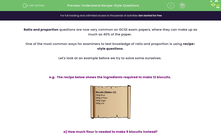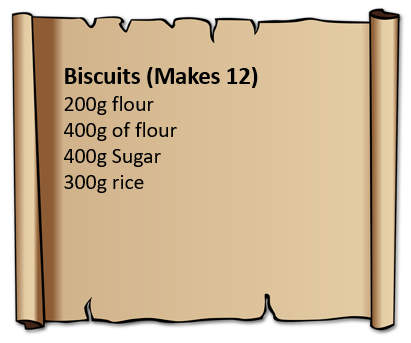# Understand Recipe-Style Questions

In this worksheet, students will practise solving problems involving recipe style questions.Key stage:  KS 4

Year:  GCSE

GCSE Subjects:   Maths

GCSE Boards:   AQA, Eduqas, Pearson Edexcel, OCR,

Curriculum topic:   Ratio, Proportion and Rates of Change

Curriculum subtopic:   Ratio, Proportion and Rates of Change Direct and Inverse Proportion

Difficulty level:#### Worksheet Overview

Ratio and proportion questions are now very common on GCSE exam papers, where they can make up as much as 40% of the paper.

One of the most common ways for examiners to test knowledge of ratio and proportion is using recipe-style questions.

Let's look at an example before we try to solve some ourselves.

e.g.  The recipe below shows the ingredients required to make 12 biscuits.a) How much flour is needed to make 9 biscuits instead?

Firstly, we need to find the Highest Common Factor (HCF) of 12 and 9.

This is 3.

We now to work out how much flour is needed for 3 biscuits:

12 biscuits = 200 g of flour

3 biscuits = 50 g of flour (200 ÷ 4)

As we now know how much flour is needed for 3 biscuits, we can multiply this by three to find the amount needed for 9:

9 biscuits = 150 g of flour (50 × 3)

So 150 g of flour is needed to make 9 biscuits using this recipe.

b) How many biscuits can we make if we have 1 kg of flour and plenty of each of the other ingredients?

In this question, the amount of flour we have is what will limit the amount of biscuits we can make.

We have 1 kg or 1000 g of flour.

Each batch of 12 biscuits needs 400 g of flour:

1000 ÷ 400 = 2.5 batches of biscuits

1 batch = 12 biscuits so 2.5 batches = 12 × 2.5 = 30 biscuits

So 30 biscuits can be made according to this recipe and using 1 kg of flour.

In this activity, we will apply our knowledge of ratio and proportion in recipe-style questions, where we may be asked to find the value of an ingredient required to make an alternative amount or to find how many can be made with a different amount of one ingredient.

### What is EdPlace?

We're your National Curriculum aligned online education content provider helping each child succeed in English, maths and science from year 1 to GCSE. With an EdPlace account you’ll be able to track and measure progress, helping each child achieve their best. We build confidence and attainment by personalising each child’s learning at a level that suits them.

Get started